## Wednesday, October 27, 2021

### Light Independent Reactions

Biology Index

Where are we going with this? The information on this page should increase understanding related to this standard:  Understand how photosynthesis turns light energy into chemical energy.

Article includes ideas, images, and content from Troy Smigielski (2021-10)

Light Independent Reactions
(…and then… BAM! Magic!)

The photosynthesis process includes two distinct steps. The light dependent reactions and the light independent reactions. The net result of photosynthesis is the production of oxygen that is released and sugar that is stored to be used by the plant.

The light dependent reactions produce oxygen that gets released by the plant as a byproduct. It also produces ATP and the electron carrier NADPH which are used in the Light Independent Reactions.

Keep in mind that the oxygen produced in the light dependent reactions is NOT needed by the plant. It is a byproduct that is given off.

The goal of LDR is to create energy rich molecules for the Calvin cycle to use.

The light independent reactions are also called the Calvin cycle or dark reactions. These reactions take place in the stroma and do not require light.

The dark reactions use three reactants to produce the final product (sugar).

CO2 (Carbon dioxide)

The Calvin Cycle uses the ATP and the NADPH made in the light dependent reactions for energy to fuel itself.

In the process, the ATP (plus water, H2O) is broken into ADP (Adenosine diphosphate, C10H15N5O10P2) and a phosphate ion (PO4-3)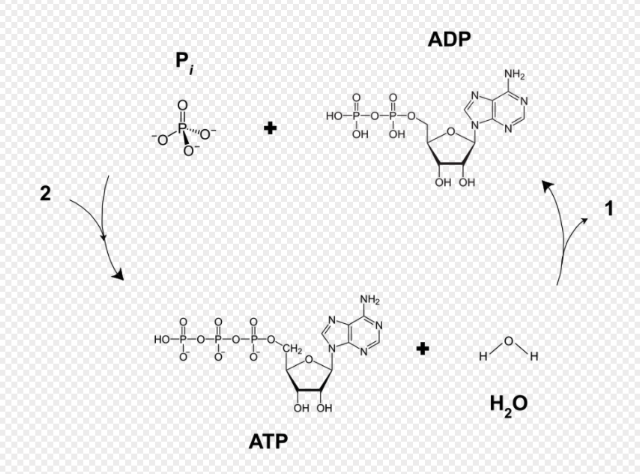Source, 2021-10

Whereas the following represents the reaction going on in the diagram…

C10H16N5O13P3  +  H2O --> C10H15N5O10P2  +  PO4-3

…a close look reveals that there are more hydrogen atoms on the reactant side. Remember that the ATP-ADP cycle is only PART of what is going on!

So, here's the big idea…

ATP + Water becomes ADP + phosphate + ENERGY! ENERGY! ENERGY! (and also some H atoms)

The Calvin Cycle also uses the CO2 that we exhale to make glucose.

Recall that the CO2 comes into the plant from the atmosphere. It enters the leaves through openings called stomata. Stomata are protected by guard cells that open during the day and close at night.

The Calvin Cycle Steps

So… there's a reason its called a cycle! It goes around and around as it produces the sugar. The process can be understood only by seeing it in motion. There's not exactly a starting place; all of the cycle parts are present when…

Okay, let's start with a CO2 coming in… what happens to it?

Step 1: One CO2 molecule enters the leaf. It combines with a 5-Carbon molecule called RuBP.

Step 2: The 6-Carbon molecule splits into two 3-Carbon molecules called 3-PGA (PGA).

Step 3: ATP and NADPH (high energy) from light dependent reactions are used to convert the PGA into G3P (aka PGAL). When ATP is used for energy, it becomes ADP.

When NADPH is used for energy, it becomes NADP+. These compounds go back (eventually) to the light reaction to be reenergized.

Step 4: One carbon from the G3P molecules goes on to start making a glucose. The other 5 carbons of the G3P are regenerated into RuBP to start the cycle over again.

Step 5: The cycle repeats 6 times to form one molecule of glucose.

The above diagram shows the reactants and products of the cycle happening 3 times (all in the same diagram). It has to happen 6 times to produce one glucose molecule. Each cycle adds one carbon to what will become the glucose chain (at the bottom).

Some diagrams you might see will show this cycle differently.

The above diagram shows the cycle after it has happened 6 times.

Light Independent Reactions: Bottom Line

1. Light independent reactions are also called the Calvin Cycle and dark reactions.

2. The Calvin Cycle happens in the stroma of a chloroplast.

3. CO2 from the atmosphere is used to start the Calvin Cycle.

4. Energy from the light dependent reactions is used in the light independent reactions.

6. After 6 rounds of the Calvin Cycle, one glucose molecule is made.

Light reactions: Makes energy for the Calvin Cycle
• Requires water
• Makes ATP and NADPH for Calvin Cycle
• Releases oxygen.
Dark reactions: Makes glucose for other organisms
Requires carbon dioxide
Releases glucose.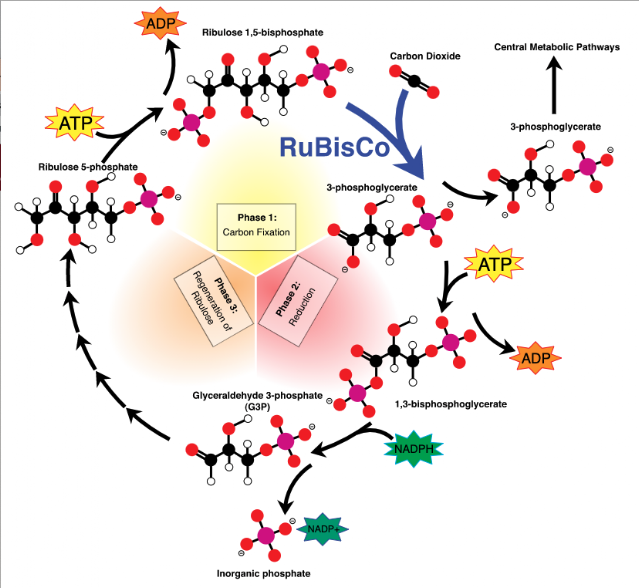Source 2021-11-01

## Monday, October 25, 2021

### Projectile Motion When Vertical Displacement Is Not Zero

Where are we going with this? The information on this page relates to the skills needed to investigate and evaluate the graphical and mathematical relationship (using either manual graphing or computers) of one-dimensional kinematic parameters (distance, displacement, speed, velocity, acceleration) with respect to an object's position, direction of motion, and time.

Projectile Motion
When Vertical Displacement Is Not Zero
Why is this even a thing!Source 2021-10-25

What happens if a projectile is set into motion, but from a position above the horizontal? Like in shot putting.

The shot is launched from a distance of around 2.25 meters above the ground. How does this change the calculations?

The problem starts out just as if the projectile began on the ground.

The difference occurs in step 4 (below). Because the vertical displacement is NOT ZERO, the projectile has further to go before hitting the ground.

tdown is now NOT EQUAL to tup.

Keep in mind that you COULD use this method, even if the initial distance is zero. You would just get the same number for time up and time down.

With that in mind, let's get going…

______________________________

STEP 1: Draw and Label

STEP 2: Find the y and x components of the velocity v where the angle is θ.

v • cosθ = vx
v • sinθ = vyi

Whereas the velocity up/down will change due to gravity, it will carry the subscript i for "initial."

Whereas we are ignoring air resistance, vis a constant.

STEP 3: Find time up:

To find time up, use the velocity equation…

v= vi + at

Plug in for the specific situation…

0 = vyi + (-9.81)tup
-vyi = -9.81•tup
-vyi /-9.81 = tup
vyi /9.81 = tup

Find time down:

To find tdown, you will need to use the distance equation:

df = di + vit + 1/2at2

The most accurate way to think about this is to say df is the ground and di is the total vertical displacement, dy(max) which is how much the projectile went up (∆dy) plus the initial vertical displacement (dyi). Since these would be measured from the ground to the top of the trajectory, they would be positive meaning a is in the opposite direction and is negative. Further df would be when it hits the ground and would be zero.

Did you notice that, to find time down, we have to find max vertical displacement (which we'll call dy(max))?

To do that, we will ALSO use the distance equation, but with the value for tup which we found in Step 3.

STEP 4: Find how high it goes.

We use that value, tup, in the distance equation to find how high it goes, dy(max).
df = di + vit + 1/2at2

Plug in for specific case:

dy(max) =  dyi + vyi•tup + 1/2at2

STEP 5 Find time down

Now that we know how high it went, we can use the distance equation  AGAIN to find tdown with the following values.

df = 0 m (the ground)
di  = dy(max)
v =  0 m/s (because we are measuring from when it stopped going up, max distance in y direction)
a = g = -9.81 m/s/s (or -9.8 m/s/s)
t =  tdown

Solving for t we find that…

df = di + vit + 1/2at2
0 = dy(max) + •  tdown  + 1/2(-9.81) tdown2
-dy(max)  =  -4.905 tdown2
-dy(max) /  -4.905 = tdown2

The negatives cancel. Take square root of both sides:

tdown = √  (dy(max)) / 4.905

STEP 6: Find the total time:

ttot = tup + tdown

STEP 7: Find the horizontal displacement, dx:

Whereas…

di  = dxi = 0
v =  vx   (From Step 2)
a = 0
t =  ttot

df = di + vit + 1/2at2
dx = 0 + vxttot + 0
dx = vxttot

______________________________

By now, you will have noticed that there are a lot of steps and a lot to understand.

Conceptually, it's not too hard:

The horizontal distance is how far it goes before it hits the ground, and is found easily:

dx = vxttot

"Before it hits the ground" (ttot) is how long it takes to go up added to how long it takes to come back down.

It is in the most complicated steps (3 and 4) that we find the "how long it takes".

Conceptually, it might help to partition off parts of your paper to do a few things…

1. Draw it out and find vx and vyi…
2. Make a box in which you will find dx (at the end of the process)
3. Figure out the time up and time down then go back to the box…

Once you get through the process conceptually a few times, you can fall into a "calculator" process (though you'll still need to write down some values as you go." Consider the checklist that follows…

CHECKLIST/SUMMARY

So, if you are given theta and the velocity, here's a checklist sort of process…

• #1 Draw the diagram and label everything.
• #2 Find the component velocities in the x and y directions:
v • cosθ = vx
v • sinθ = vyi

• #3 Find the time up using vy and acceleration due to gravity (probably 9.81 m/s/s)
tup = vyi  / 9.81

Find the time down:

• #4 Find how high it goes

• First, find dy(max):
dy(max) =  dyi + vyi • tup + 1/2•(-9.81)•tup2

• #5 Find time down
• Then, find tdown:
tdown = √  dy(max) / 4.905

• #6 Find the total time where…
ttot = tup + tdown

• #7 Use the distance equation to find the horizontal displacement:

d= vxttot

where vx was found in step 2 and was found in step 4.

Something like this…The scribbling above follows the steps without labeling them with numbers. Go down the left, the back up and down the right.

CHECKLIST/SUMMARY

So, if you are given theta and the velocity, here's a checklist sort of process…

• #1 Draw the diagram and label everything.
• #2 Find the component velocities in the x and y directions:
v • cosθ = vx
v • sinθ = vyi

• #3 Find the time up using vy and acceleration due to gravity (probably 9.81 m/s/s)
tupvyi  / 9.81

Find the time down:

• #4 Find how high it goes

• First, find dy(max):
dy(max) =  dyi + vyi • tup + 1/2•(-9.81)•tup2

• #5 Find time down
• Then, find tdown:
tdown = √  dy(max) / 4.905

• #6 Find the total time where…
ttot = tup + tdown

• #7 Use the distance equation to find the horizontal displacement:

dvxttot

where vx was found in step 2 and ttot was found in step 4.

## Friday, October 22, 2021

### Light Dependent Reactions

Biology Index

Where are we going with this? The information on this page should increase understanding related to this standard:  Understand how photosynthesis turns light energy into chemical energy.

Article includes ideas, images, and content from Troy Smigielski (2021-10)

Light Dependent Reactions
(So, explaining the magic?)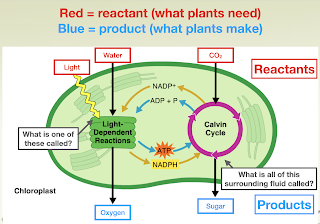The photosynthesis process includes two distinct steps. The light dependent reactions (LDR) and the light independent reactions. The net result of photosynthesis is the production of oxygen that is released and sugar that is stored to be used by the plant.

The LDR produce oxygen that gets released by the plant as a byproduct. This is the oxygen we breathe, but it is not our only source of oxygen. There are phytoplankton in the ocean that produce about 50% of our oxygen.

While it is very nice of plants to produce oxygen that we can breath, oxygen is actually a byproduct… it is something that just happens to be formed; something the plant actually does not need.

The main function of the light dependent reactions is to make energy for the light independent reactions to use. Remember, photosynthesis converts light energy into chemical energy. The light dependent reactions is the first step in creating the chemical energy.

The energy of cellular functioning is called adenosine triphosphate
(ATP). ATP is a fairly complex molecule in which is a chain of three phosphates. The "links" in the chain are chemical bonds, and it is in the bonds that energy is stored.

ATP is an energy-rich molecule that stores energy in the 3rd phosphate bond.

Breaking off one of the phosphates releases energy and results in one adenosine diphosphate and one phosphate.

When the energy from ATP is used, it becomes ADP because the 3rd bond has been broken off.

So, back to photosynthesis…

The plant's goal is to make sugar it can use. It uses the energy in the ATP to create sugar. So, the first step in photosynthesis is to make ATP.

The LDR convert ADP into ATP.

When an organism uses the energy in ATP, the 3rd bond is broken, which releases energy. When this happens, the molecule becomes ADP again. (ADP only has 2 phosphates.)

The LDR also convert NADP+ into NADPH. This conversion attaches an electron (energy) to the electron carrier. Remember, NADPH is the electron carrier molecule in photosynthesis.

It is important to note that when NADP+ picks up a Hydrogen (which carries an electron), it becomes NADPH. When NADPH drops off its electron, it will become NADP+.

So… Quick review…

When ADP picks up energy, it becomes ATP. Does ADP have a lot of energy? Does ATP?

ATP has a lot of energy which it releases to become ADP.

Light dependent reactions take place in the thylakoid membrane. Thylakoids are the individual flattened, membranous disks in the chloroplast. The membrane of the thylakoid is also made up of a phospholipid bilayer.

Light Reaction Steps
So, then, it's kinda like magic, right?

While it is complicated and detailed, the light dependent reactions are not magic.

Step 1: Light is absorbed into the chloroplast by pigments in photosystem II (PSII). The main pigment absorbing the light is chlorophyll.

Step 2:
The energy from the light splits up a water molecule (H2O) which provides two H+ ions, two high-energy electrons, and the oxygen we breath.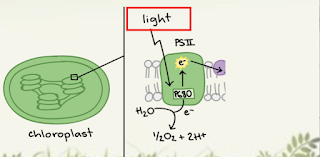As more light comes in and this continues to happen, the H+ ions start to build up inside the thylakoid membrane, so naturally they want to leave through facilitated diffusion.

To do this, they pass through a protein channel called ATP Synthase. As H+ ions flow out, ATP Synthase spins and makes ATP.

Both electrons move down the electron transport chain (ETC) into photosystem I (PSI). As they travel down the ETC, they release energy.

As electrons travel down the ETC, they release energy. This energy is used to push more H+ ions into the cell.

Step 3: Once in Photosystem I, the electrons from water receive more light energy and are attached to NADP+. This makes NADPH.

What? What? Just… what?

Light comes into PSII and chops up water into oxygen, H+ ions, and electrons.

Oxygen leaves, the H+ ions create ATP through ATP Synthase, and the electrons travel down the ETC to PSI.

Light Dependent Reactions Bottom Line
Hmm… I should probably memorize this, right?

1. LDRs happen in the thylakoid membrane and need light.

2. LDRs purpose is to make energy molecules (ATP and NADPH) for the Calvin Cycle. They also make oxygen during this process.

3. LDRs start in photosystem II and move to PS I.

LDR Application

• In the fall, plants lose chlorophyll and/or their leaves. What would happen if the plant loses chlorophyll?

• Would they be able to make oxygen in the fall?

• If there are less leaves in the winter, what happens to the carbon dioxide levels in the atmosphere?

Okay…

This is the Keeling Curve, which measures CO2 in the atmosphere. Notice the annual fluctuations.

## Wednesday, October 20, 2021

### Photosynthesis Overview

Biology Index

Where are we going with this? The information on this page should increase understanding related to this standard:  Understand how photosynthesis turns light energy into chemical energy.

Article includes ideas, images, and content from Troy Smigielski (2021-10)

Photosynthesis Overview

Before we dig into the fine points of photosynthesis, let's back up and look at two dichotomous classifications of living creatures.

An autotroph makes its own food while a heterotroph gets its food from a different source. Examples of autotrophs include grasses, trees, bushes… plants. Heterotrophs, then are all the things that eat plants or eat things that eat plants.

Let's think about this a little more scientifically… Food is energy for living organisms. And… the Law of Conservation says that energy cannot be created or destroyed. It can change form… but… It can't just show up out of nowhere.

If food is energy, and autotrophs make their own food (energy), what's going on? How do autotrophs make their own food?

Photosynthesis

"Photosynthesis is the process by which green plants and some other organisms use sunlight to synthesize foods from carbon dioxide and water. Photosynthesis in plants generally involves the green pigment chlorophyll and generates oxygen as a byproduct" (Source 2021-10)

• The main goal of photosynthesis is to build sugars (most commonly glucose).
• It uses light to create glucose and oxygen.
• In other words, photosynthesis converts light energy into chemical energy.
Using chemical notation, the reaction for photosynthesis looks like this:

6 CO2  +   6 H2O --- Sunlight ---> C6H12O6 + 6 O2
Carbon Dioxide  +   Water                                            Glucose (Energy)    Oxygen

Photosynthesis takes place in plant organelles called chloroplasts

Chloroplasts are a type of plastid, which are just a special type of organelle.Source 2021-10

• Chloroplasts have saclike photosynthetic membranes called thylakoids.
• A stack of thylakoids is called a granum.
• The fluid-like region surrounding the thylakoid membranes is called the stroma.

All chloroplasts have pigments in them that make certain colors.

The main pigment in a chloroplast is chlorophyll. It functions to absorb light energy and is located in the thylakoids. Chlorophyll is green.

The pigments that come out in the fall and produce a yellow/orange color are called carotenoids.

The Photosynthesis Process

Photosynthesis occurs in two separate sets of smaller reactions. In other words, there are two reactions in photosynthesis. One reaction will make oxygen; the other will make a sugar.

The reactions can be looked at with regard to if they require light or not. So, we have two types of reactions in photosynthesis:
• light dependent (also called the light reactions)
• light independent (also called the Calvin cycle or dark reactions)

The light dependent reactions happen first, and they require light. The light dependent reaction is the reaction that…

Creates oxygen (O2).

And it creates ATP.

NADPH is a… a… big ol' molecule. For the purposes of understanding photosynthesis, you can think of it as a molecule that can carry around electrons. Its fancy name is nicotinamide adenine dinucleotide phosphate. When it is not carrying the electron, it is written as NADP+. Since the electron has a negative charge, taking it away leave the positive (+) charge on the NADP+.  Let's stop there and get back to photosynthesis!

Light Independent Reactions require ATP and NADPH; so, Light Dependent Reactions have to happen first so that those molecules can be created.

The light independent reactions happen second, and they do not require light. These are also called the Calvin cycle or dark reactions. This is the reaction that creates the sugar, which is normally glucose.

And it uses the ATP from the light reactions.

The photosynthesis process can be thought of as a cycle of sorts. It goes sort of like this…

The light reaction begins (by taking in the reactants light, H2O, NADP+, ADP, and P).

ATP, Oxygen, NADPH are produced (and passed to the dark reaction).

The Dark reaction begins (by taking in the reactants ATP, NADPH, and CO2).

Sugar, ADP, P, and NADP+ are produced (and go back to be reused by the light dependent reactions).

More specifically, electrons are small negatively charged parts of atoms. They can take in energy and be "excited," move from atom to atom, release energy… suffice to say that they are integral parts of chemical reactions.

Electron carrier molecules are molecules that function like a taxi or energy shuttle that shuttles electrons (energy) around the cell.

The electron carrier molecule in photosynthesis is NADPH. It has an electron attached to it that is located on the hydrogen atom. (Taxi)

Do the math… If the NADP+ is missing an electron it ends up with a positive charge.

The photosynthesis reaction is, to be honest, complex. But if you focus on just the NADPH and the NADP+ you can see something. Notice how NADPH functions like a shuttle (for the electron).

In the context of photosynthesis, you can think of it like this… Electrons carry energy, which is needed in the Light Independent Reactions.

Recall the function of photosynthesis: It converts light energy into chemical energy. So these electrons provide the energy necessary to create chemical energy.

Okay… so… NADP+ goes into the light dependent reactions and comes out as NADPH. Which has picked up an electron. Where do the electrons get their energy from?

In photosynthesis, light gives these electrons their energy. This is often referred to as “light excites the electrons”.

SUMMARY:
•  Photosynthesis requires water, CO2, and light.
•  Photosynthesis makes glucose and oxygen.
•  Photosynthesis happens in the chloroplast.
•  Thylakoid = single flattened sac
•  Granum = stack of thylakoids
•  Photosynthesis has two main steps: light dependent and light independent reactions
•  NADPH is the electron carrier in photosynthesis
•  P stands for Photosynthesis
______________________________
Go deeper…

## Tuesday, October 19, 2021

Physics Index

(You're not trying to get pushy, are you?)

In physics, a force is any interaction that, when unopposed, will change the motion of an object. In other words, a force can cause an object with mass to change its velocity, i.e., to accelerate. In most situations, there are numerous forces acting on an object at any given time.

Forces are vector quantities. As such, the have magnitudes and directions.

(By the way, forces are measured in Newtons. Newtons are abbreviated as N.)

When you add up forces, you get a resultant, net force. If we agree that F is the abbreviation for forces, then…

Fnet is the net force.

We can denote other forces as A, B, C or 1, 2, 3 or something…

The net force is the vector sum of all the forces.

Since forces have both magnitude and direction, adding them requires dealing with the directions.

### Adding Force Vectors in One Dimensions

In one dimension, adding force vectors is trivial. Decide that one direction in the dimension is positive; that makes the opposite direction negative.

Let's go with left and right…

Force A is 2 N to the right
Force B is 4 N to the left
Force C is 6 N to the right

Decide which is positive and which is negative: Hmmm… let's say left is negative and right is positive

Add them up. If you get a negative for your answer the direction is left and if you get a positive for your answer, then the direction is right.

What would that actually look like…

Fnet = FA + FB + FC
Fnet = 2N + (-4N) + 6N
Fnet = 8N - 4N
Fnet = 4N

Many, many forces can be acting on an object at the same time. If they are all in the same 2 dimensional space, it is just assigning each direction a sign (positive or negative), then adding them up.

Here's an example problem you can do:

A boat is traveling up a river. The motor pushes the boat upstream with a force of 200 N. The current pushes the boat downstream with a force of 10 N. The air resistance of the moving boat creates a force of 5 N in the direction opposite the boat's movement (downstream).

What is the net force on the boat?

### Adding Force Vectors in Two Dimensions

In the real world… Well, aligning all of the forces into two dimensions is uncommon. More likely, forces will be acting on an object at different angles.

The trivial case is when all of the forces are aligned on two perpendicular axes. Let's go with Up-Down and Left-RightFancy illustration 1

In the image, A and B are perpendicular to C and D. The first thing to do is to combine the Up-Down vectors into a single resultant vector, then combine the Left-Right vectors into a single vector.

NOTE: You could have a zillion Up-Down vectors that would ALL combine into one resulting Up-Down vector. Likewise a zillion Left-Right vectors would also combine into one resulting Left-Right vector.

Now, you have two vectors that are at right angles. You can combine them using "The Triangle Method." Essentially…Fancy illustration 2

The not-as-trivial case is when the forces are in non-perpendicular directions. To even describe this, you need to start with some frame of reference. The cartesian coordinate system is a good way to start, to get our bearings regarding this.Fancy illustration 3

Okay, so, now we can get something like this…

Force A has a magnitude of 8 N and acts in a direction that is 35° counterclockwise from the positive x axis.

Sheesh… This does not seem like it will be fun!

It should be noted that the frame of reference can be anything. The cartesian coordinate system is just one that many of us are familiar with.

Going on with the discussion, let's continue…

Force A has a magnitude of 8 N and acts in a direction that is 35° counterclockwise from the positive x axis.

Force B has a magnitude of 10 N and acts in a direction that is 65° counterclockwise from the positive x axis.

So, that would look like this: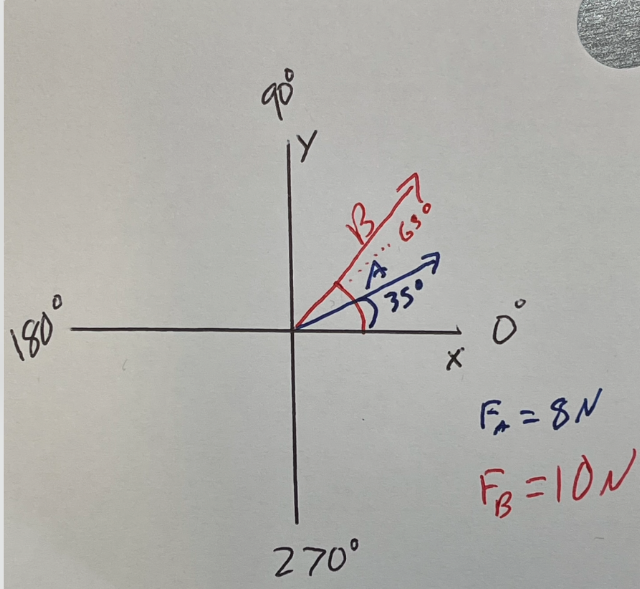Fancy illustration 4LINK
So… what is the net force, the resultant force vector? We could use the imprecise graphical
method… But, nah…

What we want to do is break apart the non-perpendicular vectors into perpendicular vectors.

If we had perpendicular component vectors, we could add them up and combine them into two new vectors.

Then, we could use the method examined above to combine the perpendicular vectors.

Let's say it like this (within the cartesian coordinate system):

Step 1: Break down all vectors into x components and y components. (This will require those trig functions won't it?) This will require using trig functions and will be demonstrated in the example that follows. (Sigh… Knew it!)

Step 2: Add all of the x components into a single resultant x vector.

Step 3: Add all of the y components into a single resultant y vector.

Step 4: Now you have two perpendicular components. Do that thing from Fancy Illustration 2 above.

Step 4a: Use the Pythagorean theorem to find the resultant magnitude.
Step 4b: Use inverse tangent to find the angle.

Okay, we should actually do that with the provided example (See Fancy illustration 4.)

Force A has a magnitude of 8 N and acts in a direction that is 35° counterclockwise from the positive x axis.

Force B has a magnitude of 10 N and acts in a direction that is 65° counterclockwise from the positive x axis.

Find the net force, Fnet

STEP 1

We need first to agree that…

FAx is the part of Force A in the x direction. FAy is the component of Force A in the y direction.

That means that, where the magnitude of A is 8 N and the angle is 35,

Sin ø = FAy / FA
Sin 35 = FAy / 8 N
8 N • Sin 35 = FAy
4.6 N = FAy

Cos ø = FAx / FA
Cos 35 = FAx / 8 N
8 • Cos 35 = FAx
6.6 N = FAx

FBx is the part of Force B in the x direction. FBy is the component of Force B in the y direction.

And that means that, where the magnitude of B is 10 N and the angle is 65,

Sin ø = FBy / FB
Sin 65 = FBy / 10 N
10 N • Sin 65 = FBy
9.1 N = FBy

Cos ø = FBx / FB
Cos 65 = FBx / 10 N
10 • Cos 65 = FBx
4.2 N = FBx

STEPS 2 and 3

At this point, we have four vectors: 2 in the x direction and 2 in the y direction. We can add them…

We need another fancy illustration!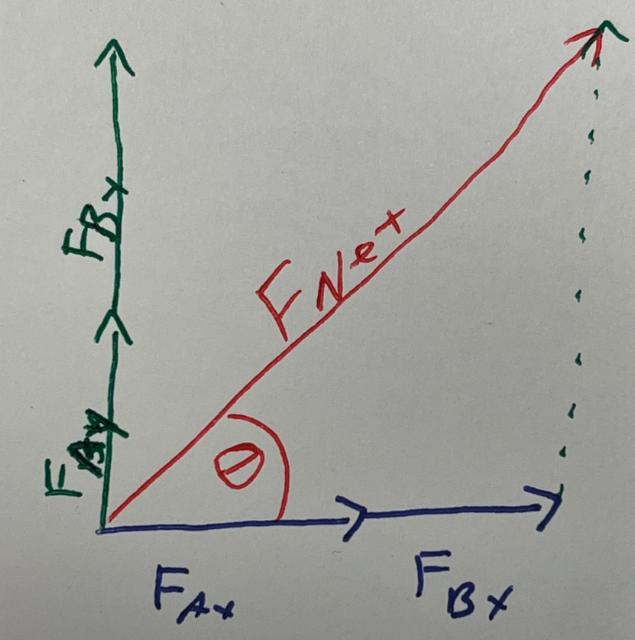Fancy illustration 5
Fx = FAx + FBx
F= 6.6 N  + 4.2 N
F= 10.8 N

F= FAy + FBy
F= 4.6 N  + 9.1 N
F= 13.7 N

STEP 4

Refer to Fancy illustration 5.

Step 4a: Use the Pythagorean theorem to find the resultant magnitude.

Fnet2 = Fx2 + Fy2

Fnet2 = 10.8 N 2 + 13.7 N 2

Fnet = 17.4 N

Step 4b:
Use inverse tangent to find the angle.

Tan øFy / Fx
ø = Tan-1 (Fy / Fx)
ø = Tan-1 (13.7 N / 10.8 N)
ø = 51.8°

Ta da! The resulting net force is 17.4 N at an angle of 51.8° counterclockwise to the x axis.